﻿ Design of Microfluidic Sensing and Transport Device

### Design of Microfluidic Sensing and Transport Device

Masoumeh Asgharighajari, Nurul Amziah, Nasri Sulaiman, Sherif Adebayo Sodeinde

Journal of Biomedical Engineering and Technology

## Design of Microfluidic Sensing and Transport Device

Masoumeh Asgharighajari1,, Nurul Amziah1, Nasri Sulaiman1, Sherif Adebayo Sodeinde2

1Department of Engineering, UPM, Serdang, Malaysia

2Department of Engineering, University of Sunderland U.K

### Abstract

This study presents detection of microfluidic droplet using an impedance measurement, study carried out with available commercial material such as copper. Model of channel and electrode, geometry a sweep from different point for sensor optimization and simulation. The study focus on admittance measurement for modeling compare to other available design with capacitive measurement. The model become more easy and in-expensive as less circuit required for electronics model. The study indicates how peak voltage can implement to measure speed of a fluid in a channel.

• Masoumeh Asgharighajari, Nurul Amziah, Nasri Sulaiman, Sherif Adebayo Sodeinde. Design of Microfluidic Sensing and Transport Device. Journal of Biomedical Engineering and Technology. Vol. 3, No. 1, 2015, pp 15-20. http://pubs.sciepub.com/jbet/3/1/3
• Asgharighajari, Masoumeh, et al. "Design of Microfluidic Sensing and Transport Device." Journal of Biomedical Engineering and Technology 3.1 (2015): 15-20.
• Asgharighajari, M. , Amziah, N. , Sulaiman, N. , & Sodeinde, S. A. (2015). Design of Microfluidic Sensing and Transport Device. Journal of Biomedical Engineering and Technology, 3(1), 15-20.
• Asgharighajari, Masoumeh, Nurul Amziah, Nasri Sulaiman, and Sherif Adebayo Sodeinde. "Design of Microfluidic Sensing and Transport Device." Journal of Biomedical Engineering and Technology 3, no. 1 (2015): 15-20.

 Import into BibTeX Import into EndNote Import into RefMan Import into RefWorks

1234
Prev Next

### 1. Introduction

Micro scale fluid manipulations in a channel has interest many researchers, microfluidic sensor device design and development as the potential to influence many field of research like biological analysis, chemical synthetics , this also include electronics design and information technology, though, the development is still consider as early stage, where there are fundamental studies of microfluidic science and development need to approach such as, fluid flow analysis, heterogeneous fluid mixing, dimension and channel optimization, material utilization, enhancement and sustainability.

However, several works had carried out for microfluidic modeling, more than a decades, microelectronic industry has been moving towards the maximum compactness possible . Drastic increase in the number of electronic components per unit volume has led to ever-increasing amounts of heat fluxes, other focus base on the use of capacitive mechanism for fluid sensing, ultrasonic transducer, electromagnetic sensor, breeze sensor, coriolis mass flow sensor, thermal transport . Another typical example is droplet detection changes in microfluidic channel using coplanar electrodes.

Microfluidic mixing also consider being useful and important to electrochemical and medical industries; microfluidic devices are often good for homogenization of reagent solutions, molecules detection, such as RNA and DNA .

In this paper, analysis on gap and channel diameter modelled and analyzes, study focus on channel geometry, fluid control and velocity profile. Therefore, two conductive plates were used while water considered as our fluid material.

Configuration of multiple electrodes around the channel examined, using this techniques allow us to measure changes in capacitance, electric potential across integrated device and hence the dielectric constant of the fluid ,

In this paper, fluid drive base on pressure fluid drive and electroosmotic effect while electric field produced between electrodes observed and sensitivity and fluid flow noticed to depend on channel geometry and potential difference.

### 2. Principle

Sensitivity of the two conductive plate sensor depend on the geometry of the device or the channel itself, for any given construction of multiple electrodes in a channel having a small gap between these multiple electrode, electric field will produced while sensitivity measurement will highly depend on channel geometry which the paper work seek to optimize in COMSOL Multiphysics simulating software.

Model of microfluidic channel offer pressure driving for fluid velocity control as unbalance motion and stoke flow equation while sensitivity of sensor is highly dependent on the geometry of the channel.

However, understanding of dielectric material properties play an essential role in this project, in this paper, Dynamics of a compressible fluid was considered; also water was examined as polar di-electric for modeling purpose. Ignore the effect of breakdown therefore, the body of force acting on a liquid and dielectric effect reduce to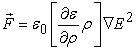(1)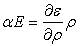(2)

The study consider flow of fluid as flow of electrons in a circuit, this assumption brought about the possibility of mapping the electric circuit elements and theory onto corresponding microﬂuidic circuit elements and models, (3) and (4) is reduced equation to support the model of the fluid toward the construction and simulation of electronics.(3)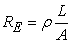(4)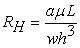(5)

Where the resistivity of the conductor, length of the conductor and cross-sectional area are ρ[Ωm], L[m] and A[m^2] respectively therefore, Ohm’s law ﬁnds its counterpart in the so called Hagen-Poiseuille’s law with deriving with pressure diﬀerence ∆p[Pa] across the microfluid channel, volumetric ﬂow rate Q[m/s]and hydraulic resistance RH[Pa · s/m^3].

### 3. Method and Material

Fluid (water) at temperature of(299.817K) was assumed with dielectric permittivity of 80 with internal resistancefor calculation and fluid modeling purpose.

Sensor modeling continue by cascading of multiple copper electrodes, droplet detection rely on dielectric property and dielectric permittivity of water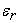at(299.817K).

Detection of droplet was possible through a coplanar electrode, that is, electrodes are placed parallel to each other inside the micro-channel. Design considers thickness of electrode (e), insulating layer thickness (t), aspect ratio (r), scaling ratio of channel width and channel height, channel height (d/r), channel width (w*r) and separation between electrodes (s).

To optimize the sensitivity, two electrodes designed as a pair called finger, four fingers are designed to improve sensitivity. Higher number of fingers, being close of electrode pairs (finger) and wider space between two fingers lead to better sensitivity.

The design include a passive layer, the space between the electrode and the insulator to prevent noise and contamination from sensor therefore, an analytical model approach by Chen et al. introduce which was given as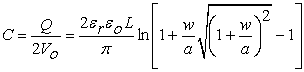(6)

Whereis the electric constant, L and W are the length and Width of single electrode respectively and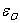is the electric constant while equation assumes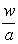>> 1.Download asVeiw figureFigures index
Figure 1. Double layer formed across the channel

Consideration of the double layer formed across the channel from two capacitance named, diffuse layer capacitance (Cs) which will arise due to excess iron or depletion in channel and also due to free electrons and independent of electrolyte is necessary because any small effect arise due to depletion can also dominate the admittance of the system or sensor, therefore, Cs can be given as(6)

Where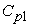=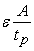(A – area of electrode top surface,–passivation layer thickness) and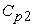is calculated (6). Model was performed at frequency domain while it was observed that frequency of the applied ac voltage, flow rate and conductivity of the fluid are the factors affecting the admittance of the fluidic system, combination of internal resistant(1),(7) and outer Helmholtz plane capacitance of the electrode,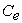the final model is given as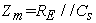(7)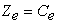(8)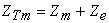(9)
3.1. Configuration of capacitive flow sensor

In this model dielectric constant value phenomenon was used, resulting electric field was varied for fixed height geometry with different width between electrodes. Distribution of electric field across electrode place in beneath inside micro-channel. Figure 2 described the electrode was placed to test against sensitivity considering the channel width, decreasing electrode spacing (width) along the channel monotonically increases sensitivity, to determine the highest signal, multiple sensor were varied as shown in Figure 3.

Configuration provide four finger of copper electrode to the channel, Thickness (t) as 250 micro-meter, Thin layer Thickness (t) as 100 micro-meter (Insulator Layer below electrode), Channel width and height as 800 and 500 micro-meter respectively with 24000 micro-meter long, separation between electrodes (s) as 100 micrometer and separation between fingers at 3400 micro-meter.Download asVeiw figureFigures index
Figure 2. Describe that, less electric field generated when electrodes are far from each otherDownload asVeiw figureFigures index
Figure 3. Describe the highest sensitive region for sensor position as electrodes are close to each other
3.2. Pressure Drive Fluid Control

Fluids controlled examine by using a microfluidic fluidic drive pressure method and droplet size examine was 500 micro-meter from both width and height, spacing between two electrode at a single finger was 400 micro-meter, the total width and long of the channel is 800 and 24000 micro-meter respectively while the total length of each electrode inside the channel is 600 micro meter per electrode. Final capacitive sensor designed for electronics purpose in order measure the speed using the resulting admittance value in correlation with total impedance and electric field.

The thicknesses of the insulator play an important role for maximization of electrode sensitivity, during the layer separation of the insulator; it was found that the peak occurs at smaller separation of the thinner layer. Insulating layer thickness held constant and the aspect ratio of the channel was also varied.

3.3. Electrical Potential Fluid Control

In this project, electroosmotic process involve the utilization of electric field to move fluid in channel in which, the EDL potential and the net charge density are related via the Poisson equation, (13). Model utilized an incompressible ﬂuid ﬂow, creeping flow or stoke flow analysis, (10), continuity equation, (11) and low Reynold number, (12) neglecting convective terms or Re<<1 .

However, model consists of inlet, outlet and open boundary condition, open boundary condition allows two way flow of fluid velocity, and it could serve either as inlet or outlet. This method is typically useful for electroosmotic fluid mixing in microchannel for microfluidics sensor design.(10)(11)(12)(13)Download asVeiw figureFigures index
Figure 4. Fluid boundary condition for electroosmotic analysis

### 4. Result and Discussion

This phase explain how the sensor design, drive fluid, detect droplet and detect fluid velocity or speed.

4.1. Detection of Droplets

Electrodes extract the droplet detection and speed moving in a micro-channel, to determine the highest and lowest signal for impedance measurement, multiple sensor vary after observing the highest impedance measurement, the admittance observe to change between 4500μm,8500μm, 13500μm and 17500μm.Download asVeiw figureFigures index
Figure 5. 3D View of electrode configuration with four finger and channel modelingDownload asVeiw figureFigures index
Figure 6. Electrical potential at finger three of configured electrode giving a value of 12.4 VAC with 12 VAC supplyDownload asVeiw figureFigures index
Figure 7. Arrow point t direction of electric field during model simulation

Water consider as fluid model with constant relative permittivity of 80, electric field change with change in charge, the greater the charge, the greater the electrified generated, admittance of the model is generated from Comsol Multiphysics Software while impedance in the model is inverse of admittance value, therefore, method used to understand the total impedance value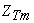is to find the admittance first and divide it by 1 in order to generate the total impedanceat each finger of the model.

Therefore, when charge increase, increase, electric field increase and cause increment in electrical potential across electrode as observe in Table 1, capacitance value base on evaluate voltage and change value, the equation provided that, capacitance is proportional to voltage and inversely proportional to change of the electrode.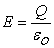(10)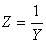(11)

While Y is admittance, Z is also, that is, the total impedance observe from each fingers, C is capacitance, C is electric potential across electrode and Q is the charge across electrode of the construction model,is the relative permittivity and E denote as electric field.

#### Table 4. Impedance, admittance, approximate distance and time taking for fluid to flow from inlet to outlet of the channel

For these measurements 500 micro –meter long droplets were used, this cover 70% of a single finger electrode. Approximately four high peak signals were observed thoroughly and with addition of peak signal which indicate the first finger with low amplification, this problem might arise due to less electric field produce within this range (Figure 6).

4.2. Electroosmotic Fluid Control

Electrical potential fluid drive control physics include, electric current analysis along with creep flow, equation, incompressible flow and steady state analysis. however, design consist of AC voltage at each of the 4 boundary as highlighted in Figure 4, according to Figure 8 result obtained, 100 volt, 0 volts,500 volts and 0 volts append to inlet, outlet, open boundary-1 and open boundary-2 of the microchannel while result directed fluid flow from inlet to open boundary-1. Figure 9 results provide the streamline of fluid mix flow and directed from inlet and open openboundary-1 to outlet using potential difference.Download asVeiw figureFigures index
Figure 8. Inlet to open boundary condition – 1 with electroosmotic fluid control analysisDownload asVeiw figureFigures index
Figure 9. Flow streamline from Inlet to outlet using electroosmotic fluid mixing and control analysis
4.3. Detection of Speed

Speed detection was achieved with rising/falling signal plotted in Figure 10 and Figure 11, Therefore, as the fluid reaches each finger, electric field increase while increase in electric field tend to cause increase in current density, these phenomenon cause increase in admittance.

Observation of changes in admittance has a direct relation with impedance since impedance is inversed of admittance. In order to determine the speed measurement, we first examine the total length for the four fingers which result to distance travel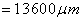, whileis the total length from first finger to last finger,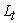is the thickness of each electrode at every finger.

Total speed is calculated with first four minimum voltage peak detected, time within this range is extracted that is, see Figure 5. Time is sum up and divided by four to determine the average speed. Since the distance travel is known, average.

Speed later determine by division of distance travel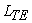by average time (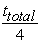). The disadvantage to this speed detection during this modeling state is that it consider that speed maintain within electrode are same speed from inlet to outlet, while further improvement related time will be discuss later in this paper work, that is, during the hardware implementation for analogue signal processing.

Measured capacitance change was between 308 micro-farad to 349 micro-farad. The analytical model suggests that increasing electrode width, changes in dielectric increasing and decreasing change in capacitance.Download asVeiw figureFigures index
Figure 10. Change in Charge vs Electric field E- It is observe that, capacitance of the system increase as there is a rise in electric fieldDownload asVeiw figureFigures index
Figure 11. Impedance result of the conductive electrode in the channel, therefore, less impedance notice at each finger (pair of electrode) of the model channelDownload asVeiw figureFigures index
Figure 12. Admittance result inverse to Impedance of the conductive electrode in the channel therefore, higher admittance notice at each finger (pair of electrode) of the model channel
4.4. Scalability and Cross Talk

The project model use a constant time measurement, this assume that the flow rate of the flow maintain constant velocity due to difficulties that encounter during channel fabrication.

The connection of external circuitry are to connect to electrode lead, that is the long beam that join each electrode together, one part of the electrode are to connect to +AC signal and second beam will lead to ground though, the circuit was design in such a way that, a resistor is connected to anode of the electrode and cathode lead to ground, the resistor serve as voltage converter.

Better sensitivity obtains by sweeping the geometrical parameters of the configured electrode in a channel. Geometry sweeping include, reducing and increasing of the thickness of the electrode, examination of the electrode dimension again the channel and investigate direction of moving electric field which find out to move toward the direction of electrode from inlet to outlet.

The project modeling design consider and test pressure drive at channel long as 10000 micro-meter to 3000 or less for fluid flow using stoke equation, a continuity equation is examined and other equation and work examine are fluid Viscosity, Reynolds number, Hagen-Poiseuille’s law and laminar flow was simulate and compare against turbulence flow also known as creep flow which the project find creep flow analysis suitable.

### 5. Conclusion

Report in this paper are the design and analytical modeling of “Design of Microfluidic Sensing and Transport Device”. Fluid flow, gap sensing and geometry has been evaluate for optimization, the sensor provide highest impedance of 3.06E-04 at 4500 micro meter inside the channel while procedure presented in this paper is applicable to most capacitive sensor design.

### Acknowledgement

I would like to thank my parents for their financial support and encouragement extending my gratitude to my supervisor (Dr Nurul Amziah) and (Sodeinde Sherif Adebayo) for his wonderful support on this project.

### References

  A.Rasmussen, C. Mavriplis, M.E. Zaghloul, O. Mikulchenko, K. Mayaram. “Simulation and optimization of a microfuidic flow sensor.” Sensors and Actuators A 88 (2001): 121-132.In article View Article  Caglar Elbuken, Tomasz, Glawdel, Danny, Chan, Carolyn L. Ren. “Detection of Micro Droplet Size and Speed Using Capacitive Sensors”, Sensors and Actuators A: Physical. Sensors and Actuators A: Physical (Elservier), 2011: 8.In article  “Droplet Operation.” Lab Chip 10, 2010.In article PubMed  F.Wang, M.A. Burns. “Multiphase Bioreaction Microsystem with Automated Onchip.” n.d.In article  George M. Whitesides. “The origins and the future of microfluidics.” 442 (2006): 1-2.In article  John Doe, Richard Miles, Richard Roe and Claus Santa. “Electroosmotic Flow (DC), Electroosmosis, Electro-osmotic ﬂow, Electrokinetic ﬂow, Electroendosmosis.” n.d. ftp://ftp.springer.de/pub/tex/latex/cyclop/sample/example.pdf (accessed April 20, 2013).In article  Meysam Rahmat. “Geometric Optimization for a Thermal Microfluidic Chip.” (Mc Grill) 2007: 109.In article  Reza Nosrati1, Mohammad Hadigol, Arian Jafari, Mehrdad Raisee and Ahamad Nourbakhs. “Numerical Investigation of Electroosmotic Mixing in Microchannels with Heterogeneous Zeta Potential.” (American Scientific Publishers) 3 (2011): 1-9.In article